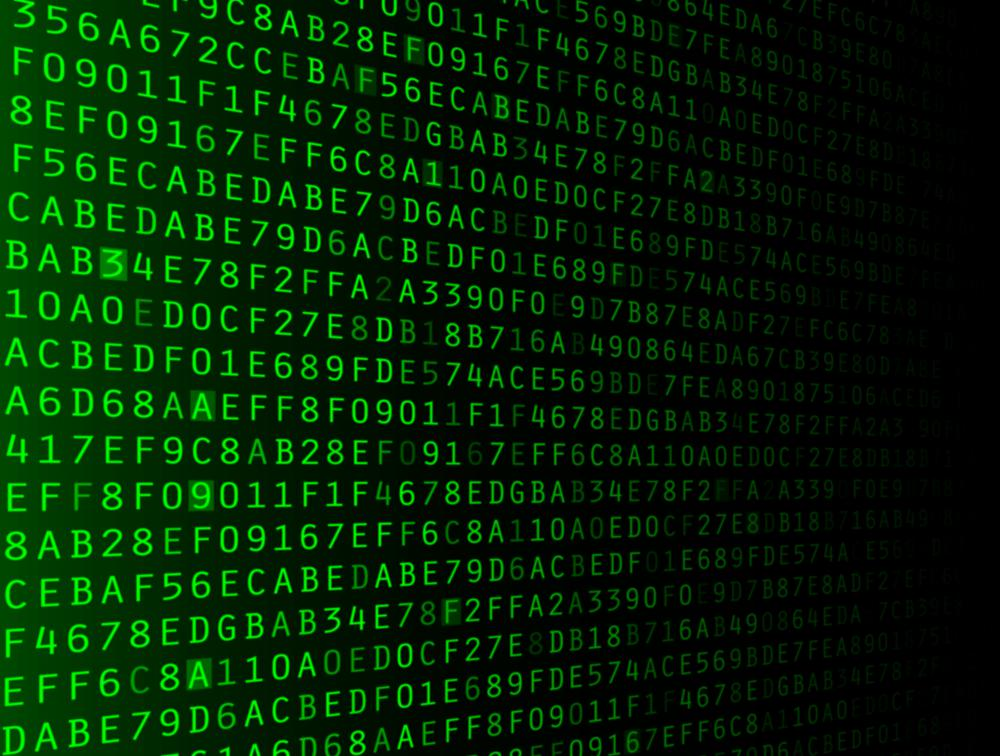# What Is an Undecidable Problem?

Mary McMahon

An undecidable problem is a question that cannot be resolved with the use of one algorithm. This is a subject of interest in mathematics and computer programming, where the undecidable problem has significant implications. Researchers with an interest in Turing machines, for example, have tackled the issue of the halting problem, looking at when computer programs stop, versus running infinitely. As with other challenges in mathematics, considerable research surrounds ways to get around undecidable problems, in addition to identifying new problems for more evaluation and study.An undecidable problem is a computing question that cannot be resolved with the use of one algorithm.

This subject involves decision problems, questions with yes or no answers. In mathematics, these are often presented in the form of formulas. A simple example might be “For any real numbers, is X evenly divisible by Y?” This is a decidable problem, because if the computer is given any values for X or Y, it can use an algorithm to answer the question. More complex problems may not be solvable with a single algorithm for all possible values.

In these cases, an algorithm might be accurate for some answers, but could be incapable of answering for other values. Given some values, the algorithm could move through a series of steps to determine whether the answer to the question was yes or no. In other cases, it wouldn’t be able to do so because it would lack the necessary information. This is a known issue with some problems involving matrices, complex analysis, and certain other functions.

Identification of an undecidable problem can occur in the context of math and computer science research. Once a problem is believed to be undecidable, researchers can apply a variety of tactics to disprove this theory. This can include developing algorithms that work for some values, discussing the specifics of the problem that make it impossible to treat effectively with an algorithm for all values, and related activities. Mathematics and computer science publications may discuss the latest progress in this field with examples of algorithms researchers have used to explore the boundaries of an undecidable problem.

Far from being a topic of theoretical interest only, the undecidable problem can have important implications for the real world. For example, some computer viruses present systems with undecidable problems. The system’s attempt to work through the problem can eat through resources, causing the system to freeze or creating system vulnerabilities. Similarly, technicians might cause a problem with a system by unwittingly presenting it with a problem it cannot solve. They might need to terminate a program or operation, which could result in data loss.

## You might also Like